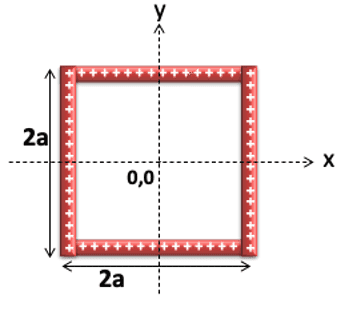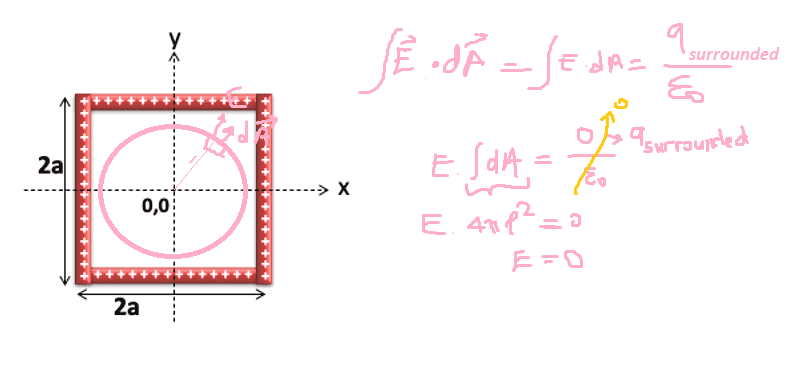# Electric field at (0,0) for this charged square conductor

requied
Homework Statement:
If this figure below is a conductor, what will be the electric field at (0,0) for the square charge distribution
Relevant Equations:
Gauss' LawCan we assume that square charge resembles a sphere shell, and think like electric field at sphere shell's center is 0.

requiedThis solution is true ? According to this,the electric field is 0 on anyplace in the square conductor.

Homework Helper
Gold Member
No this solution is not correct. You can't take the E out of the integral, as you do in the 2nd line, because the spherical gaussian surface is not symmetrical with respect to the square charge density.

Homework Helper
Gold Member
2022 Award
You are only asked for the field at (0,0). Isn't the answer obvious by symmetry?

requied
You are only asked for the field at (0,0). Isn't the answer obvious by symmetry?
Yeah it is obvious but I must attach an explain and show the calculations. Have you any offer to do it by this way? Or maybe a figure which can explain.

requied
You can't take the E out of the integral, as you do in the 2nd line, because the spherical gaussian surface is not symmetrical with respect to the square charge density.
I have a trouble with square shape symmetry. How can I come through of it? Or maybe I don't have to show calculations, just draw a figure which can explain the logic.

Homework Helper
Gold Member
I have a trouble with square shape symmetry. How can I come through of it? Or maybe I don't have to show calculations, just draw a figure which can explain the logic.
Well the truth is that this square shaped charge density doesn't offer us with any symmetry that would be useful in Gauss's law. You just can't use Gauss's law (with any possible gaussian surface) to solve for the electric field in the interior.

However the point (0,0) has a special symmetry. To see this symmetry , try to draw the electric field vectors at the center due to each of the 4 sides of the square. You ll draw 4 vectors and then you ll have to argue how they cancel out in pairs. And then you ll conclude that the E-field at the center is indeed zero.

requied
try to draw the electric field vectors at the center due to each of the 4 sides of the square. You ll draw 4 vectors and then you ll have to argue how they cancel out in pairs.
I've been thinking the same thing from the beginning. I'll solve this by this way, I hope it works for getting some point :) Thank you for attention.